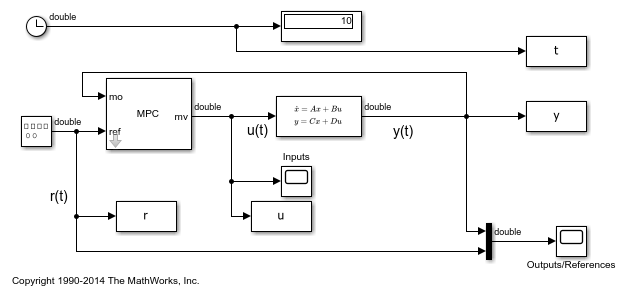Documentation

## Simulation and Code Generation Using Simulink Coder

This example shows how to simulate and generate real-time code for an MPC Controller block with Simulink Coder. Code can be generated in both single and double precisions.

### Required Products

To run this example, Simulink® and Simulink® Coder™ are required.

```if ~mpcchecktoolboxinstalled('simulink') disp('Simulink(R) is required to run this example.') return end if ~mpcchecktoolboxinstalled('simulinkcoder') disp('Simulink(R) Coder(TM) is required to run this example.'); return end ```

### Setup Environment

You must have write-permission to generate the relevant files and the executable. So, before starting simulation and code generation, change the current directory to a temporary directory.

```cwd = pwd; tmpdir = tempname; mkdir(tmpdir); cd(tmpdir); ```

### Define Plant Model and MPC Controller

Define a SISO plant.

```plant = ss(tf([3 1],[1 0.6 1])); ```

Define the MPC controller for the plant.

```Ts = 0.1; %Sampling time p = 10; %Prediction horizon m = 2; %Control horizon Weights = struct('MV',0,'MVRate',0.01,'OV',1); % Weights MV = struct('Min',-Inf,'Max',Inf,'RateMin',-100,'RateMax',100); % Input constraints OV = struct('Min',-2,'Max',2); % Output constraints mpcobj = mpc(plant,Ts,p,m,Weights,MV,OV); ```

### Simulate and Generate Code in Double-Precision

By default, MPC Controller blocks use double-precision in simulation and code generation.

Simulate the model in Simulink.

```mdl1 = 'mpc_rtwdemo'; open_system(mdl1) sim(mdl1) ```
```-->Converting model to discrete time. -->Assuming output disturbance added to measured output channel #1 is integrated white noise. -->The "Model.Noise" property of the "mpc" object is empty. Assuming white noise on each measured output channel. ```The controller effort and the plant output are saved into base workspace as variables u and y, respectively.

Build the model with the `rtwbuild` command.

```disp('Generating C code... Please wait until it finishes.') set_param(mdl1,'RTWVerbose','off') rtwbuild(mdl1); ```
```Generating C code... Please wait until it finishes. ### Starting build procedure for model: mpc_rtwdemo ### Successful completion of build procedure for model: mpc_rtwdemo ```

On a Windows system, an executable file named "mpc_rtwdemo.exe" appears in the temporary directory after the build process finishes.

Run the executable.

```if ispc disp('Running executable...') status = system(mdl1); else disp('The example only runs the executable on Windows system.') end ```
```The example only runs the executable on Windows system. ```

After the executable completes successfully (status=0), a data file named "mpc_rtwdemo.mat" appears in the temporary directory.

Compare the responses from the generated code (rt_u and rt_y) with the responses from the previous simulation in Simulink (u and y).They are numerically equal.

### Simulate and Generate Code in Single-Precision

You can also configure the MPC block to use single-precision in simulation and code generation.

```mdl2 = 'mpc_rtwdemo_single'; open_system(mdl2) ```To do that, open the MPC block dialog and select "single" as the "output data type" at the bottom of the dialog.

```open_system([mdl2 '/MPC Controller']) ```

Simulate the model in Simulink.

```close_system([mdl2 '/MPC Controller']) sim(mdl2) ```

The controller effort and the plant output are saved into base workspace as variables u1 and y1, respectively.

Build the model with the `rtwbuild` command.

```disp('Generating C code... Please wait until it finishes.') set_param(mdl2,'RTWVerbose','off') rtwbuild(mdl2); ```
```Generating C code... Please wait until it finishes. ### Starting build procedure for model: mpc_rtwdemo_single ### Successful completion of build procedure for model: mpc_rtwdemo_single ```

On a Windows system, an executable file named "mpc_rtwdemo_single.exe" appears in the temporary directory after the build process finishes.

Run the executable.

```if ispc disp('Running executable...') status = system(mdl2); else disp('The example only runs the executable on Windows system.') end ```
```The example only runs the executable on Windows system. ```

After the executable completes successfully (status=0), a data file named "mpc_rtwdemo_single.mat" appears in the temporary directory.

Compare the responses from the generated code (rt_u1 and rt_y1) with the responses from the previous simulation in Simulink (u1 and y1).They are numerically equal.

Close the Simulink model.

```bdclose(mdl1) bdclose(mdl2) ```
```cd(cwd) ```

## Related Topics

#### Implementing an Adaptive Cruise Controller with Simulink

Download technical paper